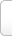﻿ 据的多音字组词_有哪些_据的多音字怎么组词_是什么读音_拼音查询

# 据的多音字组词有哪些

“据” 字共有 1 个读音：  【

## 读音为【jù】 据的多音字怎么组词

• 案据
• 霸据
• 被甲据鞍
• 本据
• 逼据
• 笔据
• 辩据
• 播越失据
• 不足为据
• 簿据
• 查无实据
• 酬据
• 窜据
• 篡据
• 大数据分析
• 大数据时代
• 单据
• 叨据
• 蹈据
• 盗据
• 道理论据
• 敌后抗日根据地
• 砥据
• 地理数据
• 地理数据库
• 典据
• 电子数据
• 电子证据
• 定据
• 扼据
• 二重证据法
• 藩镇割据
• 反据
• 方言据
• 非据
• 封建割据
• 割据
• 根据
• 根据地
• 公据
• 轨据
• 疾据
• 借据
• 进退失据
• 进退亡据
• 进退无据
• 晋冀鲁豫抗日根据地
• 晋绥抗日根据地
• 经据
• 井冈山革命根据地
• 据鞍
• 据傲
• 据比
• 据常
• 据称
• 据床
• 据此
• 据地
• 据点
• 据凡
• 据高临下
• 据古
• 据蒺
• 据经
• 据经引传
• 据理
• 据理力争
• 据梁
• 据旅
• 据乱世
• 据慢
• 据凭
• 据窃
• 据实
• 据式
• 据恃
• 据轼
• 据守
• 据说
• 据图刎首
• 据为己有
• 据闻
• 据卧
• 据梧
• 据悉
• 据险
• 据信
• 据虚
• 据依
• 据义履方
• 据有
• 据掌
• 据杖
• 据争
• 据证
• 据重
• 抗日根据地
• 考据
• 考据学
• 可据
• 跨据
• 理据
• 刘据
• 论据
• 马援据鞍
• 明据
• 盘据
• 蟠结根据
• 蟠据
• 蟠据固结
• 偏据
• 票据
• 票据(ｐｉàｏ-)
• 票据存款
• 票据交换所
• 票据交换所(票ｐｉàｏ)
• 凭几据杖
• 凭据
• 跂据
• 契据
• 欠据
• 碻据
• 窃据
• 侵据
• 确据
• 鹊巢鸠据
• 人据
• 日据时代
• 阮生据案
• 陕甘革命根据地
• 失据
• 实据
• 实验数据
• 事实论据
• 收据
• 数据
• 数据包
• 数据采集
• 数据仓库
• 数据分析
• 数据库
• 数据库管理系统
• 数据流计算机
• 数据时代
• 数据素养
• 数据通讯
• 数据元素
• 数据治理
• 碎据
• 饕据
• 讨据
• 条据
• 抟据
• 吞据
• 屯据
• 无定据
• 无据
• 无凭据
• 无凭无据
• 无线数据通信
• 袭据
• 湘鄂赣革命根据地
• 湘鄂西革命根据地
• 湘赣革命根据地
• 写据
• 信据
• 雄据
• 熊据虎踤
• 讯据
• 言必有据
• 言之有据
• 依据
• 义据
• 引经据典
• 引经据古
• 引经据礼
• 引据
• 应付票据
• 应收票据
• 拥据
• 有凭有据
• 元数据
• 援据
• 约据
• 赃据
• 凿凿有据
• 粘据
• 占据
• 真凭实据
• 镇据
• 征据
• 证据
• 证据调查
• 证据链
• 证据确凿
• 支策据梧
• 执据
• 指据
• 中据
• 中央革命根据地
• 中央革命根据地第二次反围剿”
• 中央革命根据地第四次反围剿”
• 中央革命根据地第五次反围剿”
• 中央革命根据地第一次反围剿”
• 衷据
• 专据
• 准据
• 灼据
• 资据
• 字据
• 左右江革命根据地Tip:SCCG